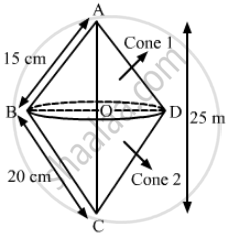Advertisement Remove all ads

# A Right Triangle Whose Sides Are 15 Cm and 20 Cm (Other than Hypotenuse), is Made to Revolve About Its Hypotenuse. Find the Volume and Surface Area of the - Mathematics

Sum

A right triangle whose sides are 15 cm and 20 cm (other than hypotenuse), is made to revolve about its hypotenuse. Find the volume and surface area of the double cone so formed. (Choose value of π as found appropriate)

Advertisement Remove all ads

#### SolutionWe have,

In ΔABC, ∠B = 90° , AB = l=15 cm and BC = l= 20 cm

Let OD = OB = r , AO = hand CO = h

Using Pythagoras therom,

"AC" = sqrt("AB"^2 + "BC"^2)

=sqrt(15^2 + 20^2)

= sqrt(225 + 400)

= sqrt(625)

⇒ h = 25 cm

As, ar(ΔABC) = 1/2xxACxxBO=1/2xxABxxBC

⇒ AC × BO = AB × BC

⇒ 25r = 15 × 20

rArr r = (15xx20)/25

⇒ r = 12 cm

Now,

Volume of the double cone so formed = Volume of cone 1 +Volume of cone 2

= 1/3 pir^2h_1 + 1/3pir^2h_2

= 1/3 pir^2 ("h"_1 + "h"_2)

= 1/3pi"r"^2"h"

= 1/3xx3.14xx12xx12xx25

= 3768 cm

Also,

surace area of the solid so formed = CAS of cone 1 + CSA of cone 2

= πrl1 + πrl2

= πr ( l1 + l2 )

= 22/7xx12xx(15+20)

=22/7xx12xx35

= 1320 cm

Is there an error in this question or solution?
Advertisement Remove all ads

#### APPEARS IN

RS Aggarwal Secondary School Class 10 Maths
Chapter 19 Volume and Surface Area of Solids
Exercise 19B | Q 34 | Page 900
Advertisement Remove all ads

#### Video TutorialsVIEW ALL 

Advertisement Remove all ads
Share
Notifications

View all notifications

Forgot password?
Course